# Michaelis Menten Equation

| Home | | Biopharmaceutics and Pharmacokinetics |

## Chapter: Biopharmaceutics and Pharmacokinetics : Nonlinear Pharmacokinetics

The kinetics of capacity-limited or saturable processes is best described by Michaelis.

MICHAELIS MENTEN EQUATION

The kinetics of capacity-limited or saturable processes is best described by Michaelis-

Menten equation: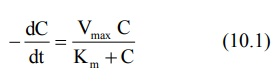Where,

–dC/dt = rate of decline of drug concentration with time,

Vmax = theoretical maximum rate of the process, and

Km = Michaelis constant.

Three situations can now be considered depending upon the values of Km and C:

1. When Km = C

Under this situation, the equation 10.1 reduces to: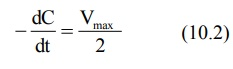i.e. the rate of process is equal to one-half its maximum rate (Fig. 10.1).Fig. 10.1 A plot of Michaelis-Menten equation (elimination rate dC/dt versus concentration C). Initially, the rate increases linearly (first-order) with concentration, becomes mixed-order at higher concentration and then reaches maximum (Vmax) beyond which it proceeds at a constant rate (zero-order).

2. When Km >> C

Here, Km + C Km and the equation 10.1 reduces to: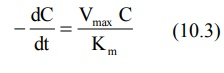The above equation is identical to the one that describes first-order elimination of a drug where Vmax/Km = KE. This means that the drug concentration in the body that results from usual dosage regimens of most drugs is well below the Km of the elimination process with certain exceptions such as phenytoin and alcohol.

3. When Km << C

Under this condition, Km + C C and the equation 10.1 will become: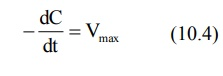The above equation is identical to the one that describes a zero-order process i.e. the rate process occurs at a constant rate Vmax and is independent of drug concentration e.g. metabolism of ethanol.

### Estimation of Km and Vmax

The parameters of capacity-limited processes like metabolism, renal tubular secretion and biliary excretion can be easily defined by assuming one-compartment kinetics for the drug and that elimination involves only a single capacity-limited process.

The parameters Km and Vmax can be assessed from the plasma concentration-time data collected after i.v. bolus administration of a drug with nonlinear elimination characteristics.

Rewriting equation 10.1.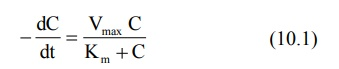Integration of above equation followed by conversion to log base 10 yields: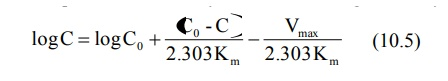A semilog plot of C versus t yields a curve with a terminal linear portion having slope –Vmax/2.303Km and when back extrapolated to time zero gives Y-intercept log BarC0 () (see Fig. 10.2). The equation that describes this line is: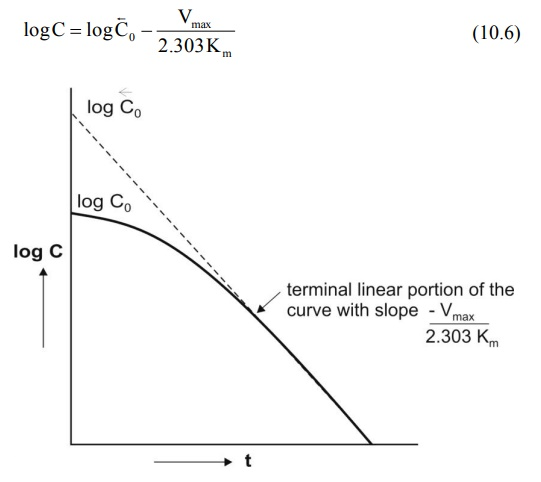Fig. 10.2 Semilog plot of a drug given as i.v. bolus with nonlinear elimination and that fits one-compartment kinetics.

At low plasma concentrations, equations 10.5 and 10.6 are identical. Equating the two and simplifying further, we get: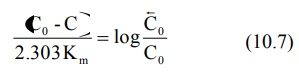Km can thus be obtained from above equation. Vmax can be computed by substituting the value of Km in the slope value.

An alternative approach of estimating Vmax and Km is determining the rate of change of plasma drug concentration at different times and using the reciprocal of the equation 10.1. Thus: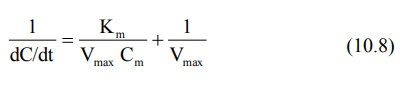where Cm = plasma concentration at midpoint of the sampling interval. A double reciprocal plot or the Lineweaver-Burke plot of 1/(dC/dt) versus 1/Cm of the above equation yields a straight line with slope = Km/Vmax and y-intercept = 1/Vmax.

A disadvantage of Lineweaver-Burke plot is that the points are clustered. More reliable plots in which the points are uniformly scattered are Hanes-Woolf plot (equation 10.9) and Woolf-Augustinsson-Hofstee plot (equation 10.10).The above equations are rearrangements of equation 10.8. Equation 10.9 is used to plot Cm/(dC/dt) versus Cm and equation 10.10 to plot dC/dt versus (dC/dt)/C m. The parameters Km and Vmax can be computed from the slopes and y-intercepts of the two plots.

### Km and Vmax from Steady-State Concentration

When a drug is administered as a constant rate i.v. infusion or in a multiple dose regimen, the steady-state concentration Css is given in terms of dosing rate DR as:

DR = CssClT                            (10.11)

where DR = Ro when the drug is administered as zero-order i.v. infusion and it is equal to FXo/τ when administered as multiple oral dosage regimen (F is fraction bioavailable, Xo is oral dose and is dosing interval).

At steady-state, the dosing rate equals rate of decline in plasma drug concentration and if the decline (elimination) is due to a single capacity-limited process (for e.g. metabolism), then;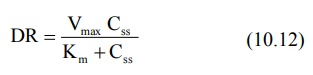A plot of Css versus DR yields a typical hockey-stick shaped curve as shown in Fig. 10.3.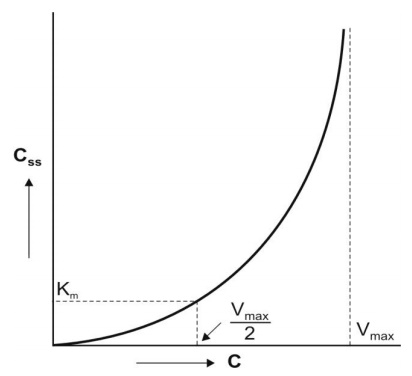Fig. 10.3 Curve for a drug with nonlinear kinetics obtained by plotting the steady-state concentration versus dosing rates.

To define the characteristics of the curve with a reasonable degree of accuracy, several measurements must be made at steady-state during dosage with different doses.

Practically, one can graphically compute Km and Vmax in 3 ways:

### 1. Lineweaver-Burke Plot/Klotz Plot

Taking reciprocal of equation 10.12, we get:Equation 10.13 is identical to equation 10.8 given earlier. A plot of 1/DR versus 1/Css yields a straight line with slope Km/Vmax and y-intercept 1/Vmax (see Fig. 10.4).Fig. 10.4 Lineweaver-Burke/Klotz plot for estimation of Km and Vmax at steady-state concentration of drug.

### 2. Direct Linear Plot

Here, the graph is considered as shown in Fig. 10.5. A pair of Css viz. Css,1 and Css,2 obtained with two different dosing rates DR1 and DR2 is plotted. The points Css,1 and DR1 are joined to form a line and a second line is obtained similarly by joining Css,2 and DR2. The point where these two lines intersect each other is extrapolated on DR axis to obtain Vmax and on x-axis to get Km.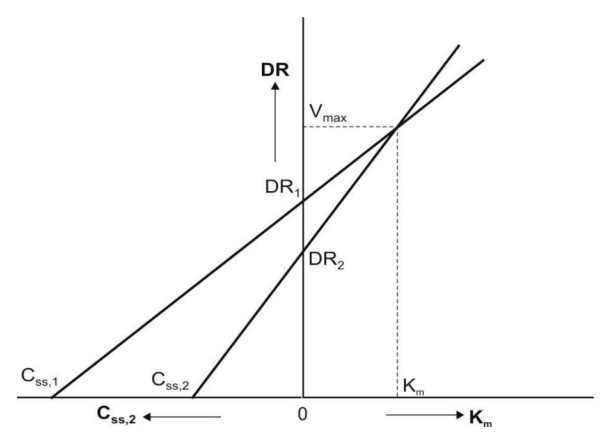Fig. 10.5 Direct linear plot for estimation of Km and Vmax at steady-state concentrations of a drug given at different dosing rates.

3. The third graphical method of estimating Km and Vmax involves rearranging equation 10.12 to yield: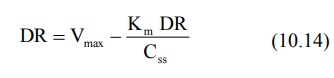A plot of DR versus DR/Css yields a straight line with slope -Km and Y-intercept Vmax.

Km and Vmax can also be calculated numerically by setting up simultaneous equations as shown below:Combination of the above two equations yields: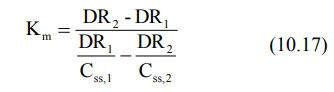After having computed Km, its subsequent substitution in any one of the two simultaneous equations will yield Vmax.

It has been observed that Km is much less variable than Vmax. Hence, if mean Km for a drug is known from an earlier study, then instead of two, a single measurement of Css at any given dosing rate is sufficient to compute Vmax.

There are several limitations of Km and Vmax estimated by assuming one-compartment system and a single capacity-limited process. More complex equations will result and the computed Km and Vmax will usually be larger when:

1. The drug is eliminated by more than one capacity-limited process.

2. The drug exhibits parallel capacity-limited and first-order elimination processes.

3. The drug follows multicompartment kinetics.

However, Km and Vmax obtained under such circumstances have little practical applications in dosage calculations.

Drugs that behave nonlinearly within the therapeutic range (for example, phenytoin shows saturable metabolism) yield less predictable results in drug therapy and possess greater potential in precipitating toxic effects.